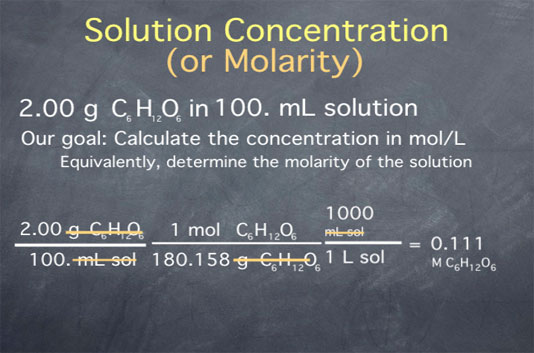# Stoichiometry Tutorials: Solution Stoichiometry

(from a complete OLI stoichiometry course)

Solution stoichiometry allows us to quantify the amount of a substance in a solution, as shown in the following video:

### Solution Stoichiometry Movie Text

Much of chemistry takes place in solution. Stoichiometry allows us to work in solution by giving us the concept of solution concentration, or molarity. Molarity is a unit that is often abbreviated as capital M. It is defined as the moles of a substance contained in one liter of solution. For instance, if a solution has a concentration of 1.20 M NaCl, this means that there are 1.20 moles of NaCl per liter of solution.

Let's begin by calculating the concentration of a solution of glucose in water. Say we take 2.00 g of glucose and add water until the final volume is 100 mL. (Glucose is a simple sugar, with a molecular formula C6H12O6.) Our goal is to calculate the concentration in moles per liter or, equivalently, to determine the molarity of the solution. We begin by writing down our initial conditions. We have 2.00 grams of glucose per 100 mL of solution.First, we convert from grams of glucose to moles of glucose. To do this, we need the molecular weight of glucose. For help calculating the molecular weight of a substance given its chemical formula, see this page. For glucose, the molecular weight is 180.158 grams per mole. We can now use this to convert from grams of glucose to moles of glucose by multiplying by one mole of glucose per 180.158 grams of glucose.

Next, we need to convert the denominator from milliliters to liters, which we do through the unit definition that there are 1000 milliliters per liter of solution. Carrying out this math, we get a value of 0.111, and canceling units, we see that the final units are moles of glucose per liter of solution. This is equivalent to 0.111 M glucose.

Note that since the experimental conditions were known to three significant figures (both the 2.00 grams and the 100 milliliters are given to three significant figures), we keep three significant figures in the final result.

The content above has been converted from Adobe Flash Player and may not display correctly.